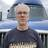Limit Input to Rational Numbers in a Text FieldThis code can be applied to "Trigger after update" in a text field to limit the input to rational numbers. It does not accept characters other than "-", [0-9] and ".", otherwise it will set the field to null. Not much can be done about the alignment unless you use the Text field as the value for a Formula field.

`"// Initialize variables for negative and leading zero";`
`"//";`
`let isNeg := "";`
`let ldZero := "";`
`"// Test for zero length string after removing non digit characters and set field to null if true";`
`"//";`
`if length(replacex(NoBS, "\D", "g", "")) = 0 then`
`NoBS := null`
`else`
`"// Test for a numeric value of zero and set field to zero if true";`
`"//";`
`if number(replacex(NoBS, "\D", "g", "")) = 0 then`
`NoBS := "0"`
`else`
`"// Test for negative, zero or more digits, decimal point, one or more digits. Set field to null if false";`
`"//";`
`if not testx(NoBS, "^-?\d*(\.\d+)?\$") then`
`NoBS := null`
`else`
`"// Test for negative and set variable if true";`
`"//";`
`if substr(NoBS, 0, 1) = "-" then`
`isNeg := "-"`
`end;`
`"// Test for decimal point in lead position. If true set leading zero variable";`
`"//";`
`if substr(replacex(NoBS, "^-?0*", "g", ""), 0, 1) = "." then`
`ldZero := "0"`
`end;`
`"// Build final string and assign to field";`
`"//";`
`NoBS := isNeg + ldZero + replacex(NoBS, "^-?0*|0+\$", "g", "")`
`end`
`end`
`end`

This will preserve numeric precision, but if you use the `number()` function with the value of the text field it will lose its precision. Btw, NoBS is the field's name in this case.An update that addresses trailing 0's in a Natural number...

`NoBS := isNeg + ldZero + if contains(NoBS, ".") then`
`replacex(NoBS, "^-?0*|0+\$", "g", "")`
`else`
`replacex(NoBS, "^-?0*", "g", "")`
`end`

replaces...

`NoBS := isNeg + ldZero + replacex(NoBS, "^-?0*|0+\$", "g", "")`Replace...

`if not testx(NoBS, "^-?\d*(\.\d+)?\$") then`

with...

`if not testx(replacex(TNum, "^-?0*|0+\$|\.\$", "g", ""), "^-?\d*(\.\d+)?\$") then`

to prevent a trailing decimal point from nullifying the input.oops, and replace...

`replacex(NoBS, "^-?0*|0+\$", "g", "")`

with...

`replacex(NoBS, "^-?0*|0+\$|\.\$", "g", "")`

An edit mode for the forum is long overdue!I think this is my final version...

`let isNeg := "";`
`let ldZero := "";`
`if length(replacex(TasN, "\D", "g", "")) = 0 then`
`TasN := null`
`else`
`if number(replacex(TasN, "\D", "g", "")) = 0 then`
`TasN := "0"`
`else`
`if not testx(replacex(TasN, "^-?0*|0+\$|\.0*\$", "g", ""), "^-?\d*(\.\d+)?\$") then`
`TasN := null`
`else`
`if substr(TasN, 0, 1) = "-" then`
`isNeg := "-"`
`end;`
`if substr(replacex(TasN, "^-?0*", "g", ""), 0, 1) = "." then`
`ldZero := "0"`
`end;`
`TasN := isNeg + ldZero + if contains(TasN, ".") then`
`replacex(TasN, "^-?0*|0+\$|\.0*\$", "g", "")`
`else`
`replacex(TasN, "^-?0*", "g", "")`
`end`
`end`
`end`
`end`

TasN is my field name.